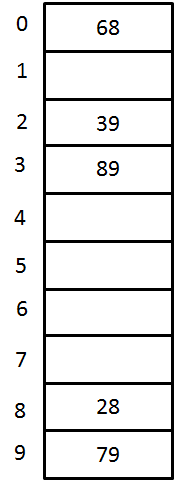# Hashing Collision resolution - Double Hashing

### <<Previous - Quadratic Probing Next - Graph>>

#### Double Hashing

Double Hashing is a hashing collision resolution technique where we use two hash functions. For double hashing, we have a hash function

hi = ( Hash(X) + F(i) ) % TableSize

where F(i)=i.hash2(X).

This F(i) will generate the sequence such as hash2(X), 2hash2(X) and so on. We use second hash function as

hash2(X) = R - (X mod R)

where R is the prime which is smaller than TableSize.

Let us consider the same example in which we choose R=7.

Solution:Fig 4. A closed Hash Table using Double Hashing

KeyHash Function h(X)IndexCollisionAlt Index
79 h0(79) = ( Hash(79) + F(0)) % 10
= ((79 % 10) + 0) % 10    = 9
9
28 h0(28) = ( Hash(28) + F(0)) % 10
= ((28 % 10) + 0) % 10     = 8
8
39 h0(39) = ( Hash(39) + F(0)) % 10
= ((39 % 10) + 0) % 10     = 9
9 first
collision
occurs
h1(39) = ( Hash(39) + F(1)) % 10
= ((39 % 10) + 1(7-(39 % 7))) % 10
= (9 + 3) % 10 =12 % 10     = 2
22
68 h0(68) = ( Hash(68) + F(0)) % 10
= ((68 % 10) + 0) % 10     = 8
8collision
occurs
h1(68) = ( Hash(68) + F(1)) % 10
= ((68 % 10) + 1(7-(68 % 7))) % 10
= (8 + 2) % 10 =10 % 10     =0
00
89 h0(89) = ( Hash(89) + F(0)) % 10
= ((89 % 10) + 0) % 10     = 9
9collision
occurs
h1(89) = ( Hash(89) + F(1)) % 10
= ((89 % 10) + 1(7-(89 % 7))) % 10 = (9 + 2) % 10 =10 % 10     = 0
0Again
collision
occurs
h2(89) = ( Hash(89) + F(2)) % 10
= ((89 % 10) + 2(7-(89 % 7))) % 10 = (9 + 4) % 10=13 % 10     =3
33

# Hashing Collision resolution - Double Hashing

### <<Previous - Quadratic Probing Next - Graph>>

#### Double Hashing

Double Hashing is a hashing collision resolution technique where we use two hash functions. For double hashing, we have a hash function

hi = ( Hash(X) + F(i) ) % TableSize

where F(i)=i.hash2(X).

This F(i) will generate the sequence such as hash2(X), 2hash2(X) and so on. We use second hash function as

hash2(X) = R - (X mod R)

where R is the prime which is smaller than TableSize.

Let us consider the same example in which we choose R=7.

Solution:Fig 4. A closed Hash Table using Double Hashing

KeyHash Function h(X)IndexCollisionAlt Index
79 h0(79) = ( Hash(79) + F(0)) % 10
= ((79 % 10) + 0) % 10    = 9
9
28 h0(28) = ( Hash(28) + F(0)) % 10
= ((28 % 10) + 0) % 10     = 8
8
39 h0(39) = ( Hash(39) + F(0)) % 10
= ((39 % 10) + 0) % 10     = 9
9 first
collision
occurs
h1(39) = ( Hash(39) + F(1)) % 10
= ((39 % 10) + 1(7-(39 % 7))) % 10
= (9 + 3) % 10 =12 % 10     = 2
22
68 h0(68) = ( Hash(68) + F(0)) % 10
= ((68 % 10) + 0) % 10     = 8
8collision
occurs
h1(68) = ( Hash(68) + F(1)) % 10
= ((68 % 10) + 1(7-(68 % 7))) % 10
= (8 + 2) % 10 =10 % 10     =0
00
89 h0(89) = ( Hash(89) + F(0)) % 10
= ((89 % 10) + 0) % 10     = 9
9collision
occurs
h1(89) = ( Hash(89) + F(1)) % 10
= ((89 % 10) + 1(7-(89 % 7))) % 10 = (9 + 2) % 10 =10 % 10     = 0
0Again
collision
occurs
h2(89) = ( Hash(89) + F(2)) % 10
= ((89 % 10) + 2(7-(89 % 7))) % 10 = (9 + 4) % 10=13 % 10     =3
33